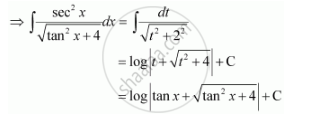Share

# Integrate the Functions (Sec^2 X)/Sqrt(Tan^2 X + 4) - CBSE (Science) Class 12 - Mathematics

ConceptIntegrals of Some Particular Functions

#### Question

Integrate the functions (sec^2 x)/sqrt(tan^2 x + 4)

#### Solution

Let tan x = t

∴ sec2x dx = dtIs there an error in this question or solution?

#### Video TutorialsVIEW ALL 

Solution Integrate the Functions (Sec^2 X)/Sqrt(Tan^2 X + 4) Concept: Integrals of Some Particular Functions.
S